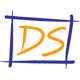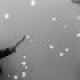# Introduction to Euclid's Geometry : Exercise 5.1 (Mathematics NCERT Class 9th)Q.1      Which of the following statements are true and which are false? Give reasons for your answers?
(i) Only one line can pass through a single point
(ii) There are an infinite number of lines which pass through two distinct points.
(iii) A terminated line can be produced indefinitely on both the sides.
(iv) If two circles are equal, then their radii are equal.
(v) In figure if AB = PQ and PQ = XY, then AB = XY.

Sol.

(i) False
Mark a point P on the plane of paper. Using a sharp pencil and a ruler, draw a line l passing through it as shown in the figure. Draw another line m passing through P. Continuing this process, we can draw as many lines as we please, each passing through point P. Thus, an infinite number of lines can be drawn passing through a given point.(ii) False
Mark two points A and B on the plane of paper. Fold the paper so that a crease passes through A. As explained in part (i) an unlimited number of creases (lines) can pass through A.Again, fold the paper so that a crease passes through B. Clearly, an unlimited number of creases (lines) can pass through B. Now fold the paper in such a way that a crease (line) passes through both A and B. We observe that there is just one crease (line) which passes through both A and B. Thus , through any two points in a plane, exactly one line can be drawn.

(iii) True
Note that what we call a line segment now a days is what Euclid's called a terminated line.In geometry, by a line, we mean the line in its totality and not a portion of it. A physical example of a perfect line is not possible.
Since a line extends indefinitely in both the directions.
So, it cannot be drawn or shown wholly on paper. In practice, only a portion of a line is drawn and arrow heads are marked at its two ends indicating that it extends indefinitely in both directions as shown.

(iv) True
On supperimposing the region bounded by one circle on the other circle if the circles coincide. Then, their centers and boundaries coincide. Therefore, their radii will be equal.

(v) True.
Because things which are equal to the same thing are equal to one another.

Q.2      Give a definition for each of the following terms. Are there other terms that need to be defined first ? What are they, and how might you define them?
(i) Parallel lines                (ii) Perpendicular lines
(iii) Line segment              (iv) Radius of a circle
(v) Square
Sol.

For the desired definition, we need the following terms :
(a) point (b) line (c) plane (d) ray (e) angle (f) circle (g) quadrilateral.
It is not possible to define first three precisely. However, a good idea of these concepts shall be given.
(a) A small dot made by a sharp pencil on a sheet paper gives an idea about a point. A point has no dimension, it has only a position.
(b) A straight crease obtained by folding a paper, a straight string pulled at its two ends, the edge of a ruler are some close examples of a geometrical line.
The basic concept about a line is that it should be straight and that it should extend in definitely in both the directions.
(c) The surface of a smooth wall or the surface of a sheet of paper are close examples of a plane.
(d) A part of line l which has only one end- point A and contains the point B is called a ray AB.(e) An angle is the union of two non- collinear rays with common initial point.
(f) A circle is the set of all those points in a plane whose distance from a fixed point remains constant. The fixed point is called the centre of the circle.
(g) A closed figure made of four line segments is called a quadrilateral.

(i) Parallel Lines : Two lines are said to be parallel when(a) They are not  intersecting (b) They are co- planar.
In figure, the two lines ${\ell _1}and\,{\ell _2}$ are parallel.

(ii) Perpendicular Lines : Two lines AB and CD lying the same plane are said to be perpendicular, if they form a right angle. We write $AB \bot CD$.(iii) Line segment : A line segment is a part of line. When two distinct points, say A and B on a line are given, then the part of this line with end- points A and B is called the line segment.It is named as $\overline {AB} .\,AB$ and BA denote the same line segment.

(iv) Radius : The distance from the centre to a point on the circle is called the radius of the circle. OP is the radius.(v) Square : A quadrilateral in which all the four angles are right angles and four sides are equal is called a square. ABCD is a square.

Q.3       Consider two 'postulates' given below :
(i) Given any two distinct points A and B, there exists a third point C which is in between A and B.

(ii) There exist at least three points that are not on the same line.
Do these postulates contain any undefined terms are these postulates consistent?
Do they follow from Euclid's postulates? Explain.
Sol.

There are several undefined terms which the students should list. They are consistent, because they deal with two different situations -
(i) says that the given two points A and B,  there is a point C lying on the line in between them ;
(ii) says that given A and B, we can take C not lying on the line through A and B.
These 'postulates' do not follow from Euclid's postulate. However, they follow from axiom stated as given two distinct  points, there is a unique line that passes through them.

Q.4     If a point C lies between two points A and B such that AC = BC, then prove that $AC = {1 \over 2}AB.$. Explain by drawing the figure.
Sol.

We have a point C lying between two points A and B such that AC = BC.
Adding AC on both sides, we haveAC + AC = AC + BC
$\Rightarrow$ 2AC = AB [Since AC + CB coincides with AB]
Therefore $AC = {1 \over 2}AB$

Q.5     In question 4, point C is called a mid- point of line segment AB. Prove that every line segment has one and only one mid-point.
Sol.        If possible, let D be another mid- point of AB.Therefore AD = DB ... (1)
But it is given that C is the mid- point of AB.
Therefore AC = CB ... (2)
Subtracting (1) from (2) we get
AC – AD = CB – DB
$\Rightarrow$ DC = – DC
$\Rightarrow$ 2DC = 0 $\Rightarrow$ DC = 0
Therefore C and D coincides.
Thus, every line segment has one and only one mid- point.

Q.6      In figure if AC = BD , then prove that AB = CD.

AC = BD ... (1) [Given]
Also AC = AB + BC ... (2) [Point B lies between A and C]
and, BD = BC + CD ... (3) [Point C lies between B and D]
Substituting for AC and BD from (2) and (3) in (1), we get -
AB + BC = BC + CD
$\Rightarrow$ AB = CD.
Thus verified.

Q.7     Why is Axiom 5, in the list of Euclid's axioms, considered a 'universal truth'? (Note that the question is not about the 5th postulate).
Sol.       Axiom 5 in the list of Euclid's axioms, is true for any thing in any part of universe so this is a universal truth.

•Anonymous

•tq

•Girish

Awesome

•Girish

Super se ooper. Bahut hi badiya

•Sir, it helped us a lot bcuz I saw ncert vedanthu I didn't understood but i can understand it now bcuz of drone study

•Ameena

Thanks a lot........it helped me a lot........

•pragnya

•Niharika acharya

Thank u so much

•Jaswant Rajput

Nice

•BHUPINDER SINGH

thnks sir

•swastik

AWESOME

•Thank you it is very helpful for me

•Himakhi rabha

It's very helpful n thank you very much

•Anjali Malik

Thanks for your help with this helps me a lot

•saranya

really good explanation . thank you so much to help me out .

•Dipu Narodia

Thanks

•Rishabh

Nice thanks bro this is very important for me

•priya chauhan

it is so much helpful for me
thank u so much

•Esha Dahiya

Thanks a lot

•Anjali suhag

Very nice thankyou

•Anjali suhag

It is very nice to do something so very thank you to

•Thanks

•Tommorow is my exam and it helps me a lot

•Shashank Varshney

Thank you sir, your solutions give me a very huge support in understanding the mathematics and also your solution help in improving my maths .

•Harsh Kaushik

It is vry help ful for me give ur app as written in solutions

•••Anonymous

•Arshdeep

Thanks a lot
Very nice explanations

•Thanks it really helped me

•Smaya willose

That's cool bro, your answers are quite simple and easy. Well, thanks for the help. OK!!!!! Bye catch you later in your next solution.

•DronStudy

Thank you 🙂

•It was really help full thanks a lot sir

•Too much helpful thanks a lot sir it helped me a lot

•Too much helpful thanks a lot sir

•Prerna

•Rajan kumar

Thanks you sir for given solutions

•Lakshit Arya

Thanks

••Thanks

Thanksu sir

•Thank u...it been helped me a lot in understanding the sums ...

•Anonymous

Wow

•pragati ...$$thanku for teaching sir....... •pragati ...$$

thanku for teaching us ......sir....

•Helped a lot

•twilight

•PRASHANT baliyan

So good ........

•Jeremie

•VERY HELP FUL..

•thanksss

•Nitasha

A verrrrrry bigggg thank uuuuu!!!!
It helps a lot in understanding the sums and also completing the homework on time...

•Samiksha garhewal

So good and very helpful keep it up

•rahul Agarwal

Really nce no tension

•Banitapa Nath

Really nice

•Banitapa Nath

It is really good & very helpful

•Bhumika khandelwal

Thankuu so muchh it's very helpful

•RISHAB K GUDIGAR

•Thank you so much

•Anonymous

•